# Exams

#### The final exam

The final exam took place Thursday, August 11 at 8:30 AM in the usual lecture Hall E. The average score of the final was 77/100 the standard deviation 17.

 The final exam Solutions

Here were some practice exams: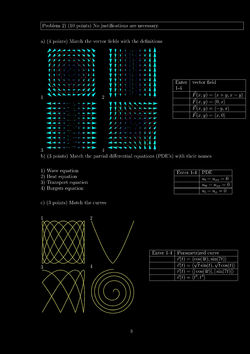Practice I, [Solutions]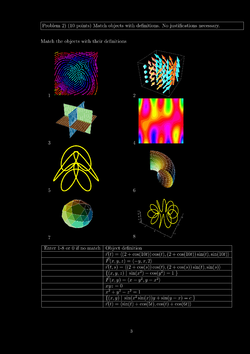Practice II, [Solutions]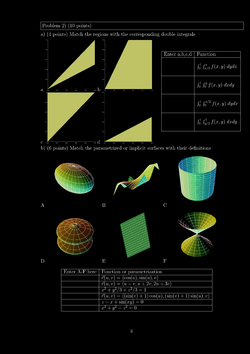Practice III, [Solutions]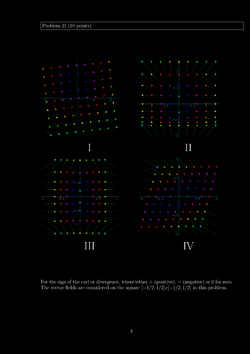Practice IV, [Solutions]Practice V, [Solutions]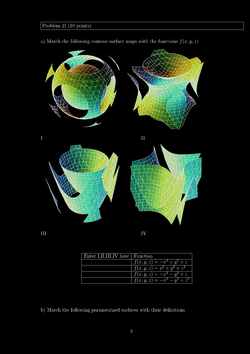Practice VI, [Solutions]
(Thanks to Alex Volkov, Brendan Lueder, XinXin Xu and Peter Haine for corrections).

#### Second hourly

The second hourly took place on July 28 2011 in lecture Hall E. We had a review on Tuesday, the 26th. The average score was 77, the standard deviation 12.
 The exam Solutions

Here were the practice exams: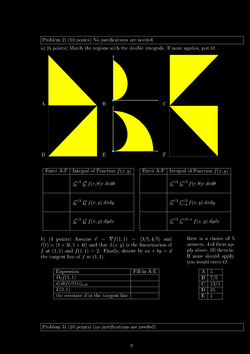Practice I, [Solutions]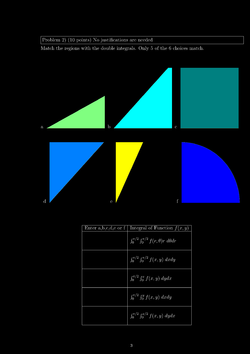Practice II, [Solutions]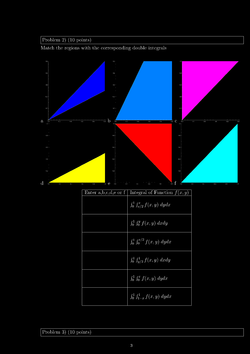Practice III, [Solutions]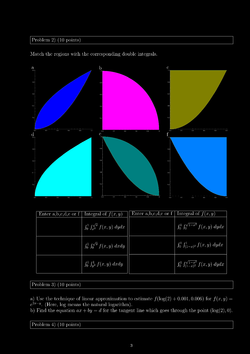Practice IV, [Solutions]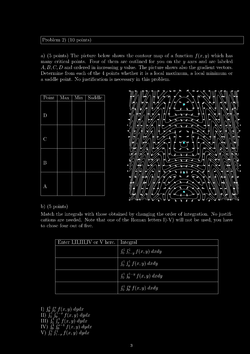Practice V, [Solutions]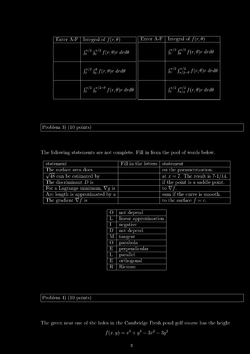Practice VI, [Solutions]

#### First hourly

The first hourly took place on July 14, 2011 at the usual place Hall E. We had a review on Tuesday, July 12 during class. Review slides. The exam will be returned on Tuesday the 19th. The average score was 78. The standard deviation 13. Here is the exam:

 The exam Solutions

(In the 11 PM draft posted 14'th, various flags in the TF were still randomly checked. The 6 AM version from the 15th was more complete. A (TF3) correction was added on July 15. Thanks to Steven Li for the alerts.) Here were the practice exams for this test: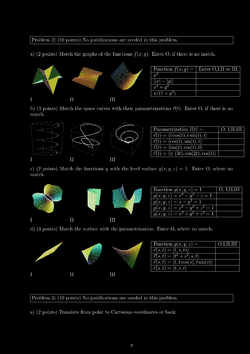Practice I, [Solutions]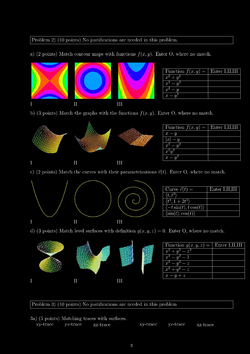Practice II, [Solutions]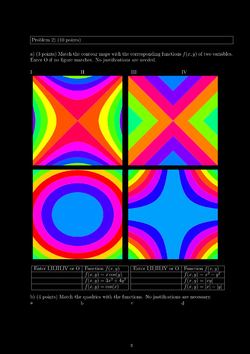Practice III, [Solutions]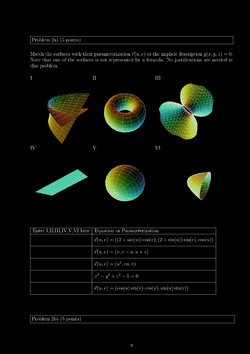Practice IV, [Solutions]